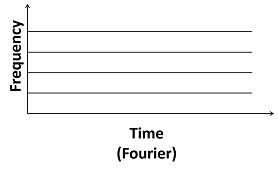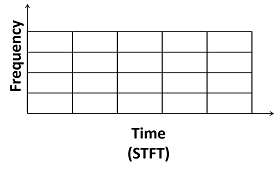Documentation

## Nonstationary Gabor Frames and the Constant-Q Transform

Nonstationary Gabor frames enable you to implement time-adaptive or frequency-adaptive analysis of signals. The functions `cqt` and `icqt` use nonstationary Gabor frames to obtain a constant-Q (frequency-adaptive) transform (CQT) of a signal. A notable strength of nonstationary Gabor frames is that they enable the construction of stable inverses, yielding perfect reconstruction.

The theory of nonstationary Gabor frames and efficient algorithms for their implementation are due to Dörfler, Holighaus, Grill, and Velasco . The algorithms in  and  implement a phase-locked version of the CQT that does not preserve the same phases that would be obtained by naïve convolution. In , Schörkhuber, Klapuri, Holighaus, and Dörfler develop efficient algorithms for the CQT and inverse CQT that do mimic the coefficients obtained by naïve convolution. The Large Time-Frequency Analysis Toolbox  provides an extensive set of algorithms for nonstationary Gabor analysis and synthesis.

In standard Gabor analysis, a window of fixed size tiles the time-frequency plane. A nonstationary Gabor frame is a collection of windowing functions of various sizes that are used to tile the time-frequency plane. Wavelet analysis tiles the time-frequency plane in a similar manner. You have the flexibility to change the sampling density in time or frequency. Nonstationary Gabor frames are useful in areas such as audio signal processing, where fixed-sized time-frequency windows are not optimal. Unlike the short-time Fourier transform, the windows used in the constant-Q transform have adaptable bandwidth and sampling density. In frequency space, the windows are centered at logarithmically spaced center frequencies.

### Decomposing the Time-Frequency Plane

The Fourier transform of f(t) is the correlation of f(t) with ej ω t:

`$F\left(\omega \right)={\int }_{-\infty }^{\infty }f\left(t\right){e}^{-j\omega t}dt.$`

Since ej ω t does not have compact support, the Fourier transform is not an ideal choice for studying nonstationary signals. If the frequency content of a signal changes over time, the Fourier transform does not capture what those changes are or when those changes occur. The partition of the time-frequency plane shown here represents this Fourier transform behavior.To perform a time-frequency analysis of a nonstationary signal, start with a real-valued even windowing function, $g\left(t\right)$, which is effectively nonzero over only a finite interval and has norm equal to one. In addition, the Fourier transform of $g\left(t\right)$ is centered at zero and is lowpass. Next, window f(t) with translates of $g\left(t\right)$. Then take the Fourier transform of the result

`$SF\left(u,\zeta \right)=\int f\left(t\right)g\left(t-u\right){e}^{-j\text{ }\zeta \text{ }t}dt.$`

Correlating f(t) with the Gabor atoms, $g\left(t-u\right){e}^{j\zeta t}$, is standard Gabor analysis. By varying u, you consider only values of f(t) near time u. The support of $g\left(t\right)$ determines the size of the neighborhood near time u. The Fourier transform of ${g}_{u,\zeta }\left(t\right)=g\left(t-u\right){e}^{\zeta t}$ is the translation by ζ of the Fourier transform of $g\left(t\right)$ and is given by

`${\stackrel{^}{g}}_{u,\zeta }\left(\omega \right)={e}^{-\left(\omega -\zeta \right)}\stackrel{^}{g}\left(\omega -\zeta \right).$`

The energy concentration of ${\stackrel{^}{g}}_{u,\zeta }\left(\omega \right)$ has variance σω and is centered at ζ. If the window, ${g}_{u,\zeta }\left(t\right)=g\left(t-u\right){e}^{\zeta t}$, shifts on a regular grid, the Fourier transform of the product of the shifted window and f(t) is the short-time Fourier transform (STFT). The STFT tiling of the time-frequency plane can be represented as a grid of boxes, each centered at (u, ζ):The set of functions $\left\{{g}_{u,\zeta }\right\}$ is known as a Gabor frame. The elements of this set are called Gabor atoms. A frame is a set of functions, {hk(t)}, that satisfy the following condition: there exist constants A > 0, B > 0 such that for any function f(t),

`$A‖f{‖}^{2}\le {\Sigma }_{k}|{|}^{2}\le B‖f{‖}^{2}.$`

The energy concentration of $g\left(t\right)$, in time, has variance σt. The energy concentration of $\stackrel{^}{g}\left(\omega \right)$, in frequency, has variance σω. The energy concentration determines how well the window localizes the signal in time and frequency. By the time-frequency uncertainty principle, there is a limit as to how well you can simultaneously localize in both time and frequency domains, as indicated by

`${\sigma }_{t}{\sigma }_{\omega }\ge \frac{1}{2}.$`

Narrowing the window in one domain results in poorer localization in the other domain. Gabor showed that the area of the window is minimal when $g\left(t\right)$ is Gaussian.

### Constant-Q Transform

In the CQT, the bandwidth and sampling density in frequency are varied. The windows are constructed and applied directly in the frequency domain. Different windows have different center frequencies and bandwidths, but the ratio of the center frequency to bandwidth remains constant. Maintaining a constant ratio implies:

• Resolution in time improves at higher frequencies.

• Resolution in frequency improves at lower frequencies.

The time shifts for each window depend on the bandwidth, due to the uncertainty principle.

The CQT depends on:

• The window functions gk are real-valued, even functions. In the frequency domain, the Fourier transform of gk is defined on the interval, [-Fs/2, Fs/2].

• The sampling rate, ζs.

• The number of bins per octave, b.

• The minimum and maximum frequencies, ζmin and ζmax.

Choose a minimum frequency ζmin and number of bins per octave b. Next, form a sequence of geometrically spaced frequencies,

ζk = ζmin × 2k/b

for k = 0,...,K where K is an integer such that ζK is the largest frequency strictly less than the Nyquist frequency ζs/2. The bandwidth at the kth frequency is set to Ωk = ζk+1k-1. Given this sampling, the ratio of the kth center frequency to the window bandwidth is independent of k:

Q = ζkk = (21/b-2-1/b)-1.

To ensure perfect reconstruction, the DC component and Nyquist frequency are prepended and appended, respectively, to the sequence.

W(ω) forms the window functions gk. W(ω) is a real-valued, even continuous function that is centered at 0, positive in the interval [-½,½], and 0 elsewhere. W(ω) is translated to each center frequency ζk then scaled. Evaluating a scaled and translated version of W(ω) yields the filter coefficients gk[m], given by

gk[m] = W((m ζs/L - ζk)/Ωk)

for m = 0, …, L-1, where L is the signal length. By default, `cqt` uses the `'hann'` window.

By the uncertainty principle, the size of the bandwidth constrains the value of the time shifts. To satisfy the frame inequality, the shift akof gk must satisfy

ak ≤ ζkk.

As mentioned previously, the window is applied in the frequency domain. The filters, gk, centered at ζk, are formed and applied to the Fourier transform of the signal. Taking the inverse transform obtains the constant-Q coefficients.

### References

 Holighaus, N., M. Dörfler, G.A. Velasco, and T. Grill. "A framework for invertible real-time constant-Q transforms." IEEE Transactions on Audio, Speech, and Language Processing. Vol. 21, No. 4, 2013, pp. 775–785.

 Velasco, G. A., N. Holighaus, M. Dörfler, and T. Grill. "Constructing an invertible constant-Q transform with nonstationary Gabor frames." In Proceedings of the 14th International Conference on Digital Audio Effects (DAFx-11). Paris, France: 2011.

 Schörkhuber, C., A. Klapuri, N. Holighaus, and M. Dörfler. "A Matlab Toolbox for Efficient Perfect Reconstruction Time-Frequency Transforms with Log-Frequency Resolution." Submitted to the AES 53rd International Conference on Semantic Audio. London, UK: 2014.

 Průša, Z., P. L. Søndergaard, N. Holighaus, C. Wiesmeyr, and P. Balazs. The Large Time-Frequency Analysis Toolbox 2.0. Sound, Music, and Motion, Lecture Notes in Computer Science 2014, pp 419-442. https://github.com/ltfat

## Related Topics

##### SupportGet trial now